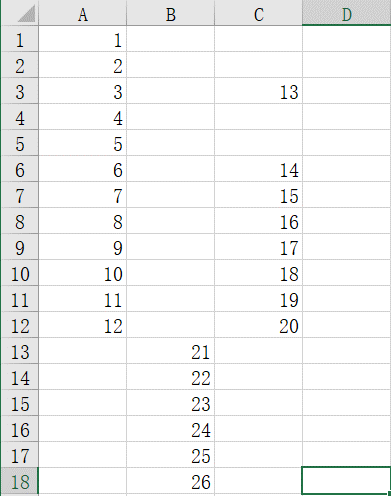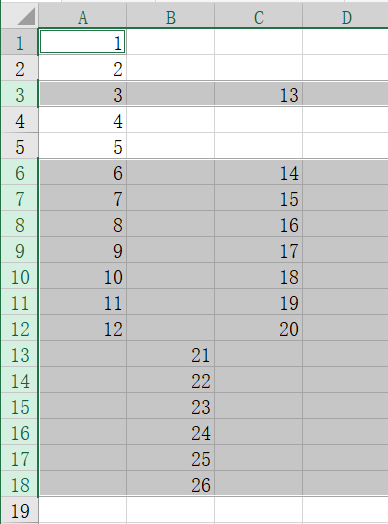• 关于VBA遍历文件夹主要用的是提供的Application.FileDialo函数来由个人进行自由选择，通过获取选择的文件夹地址之后，通过Dir函数来匹配选取文件夹下的相应的文档。相应的VBA程序代码如下： Dim sel_Path As ...
1.EXCEL VBA遍布文件夹的操作
关于VBA遍历文件夹主要用的是提供的Application.FileDialo函数来由个人进行自由选择，通过获取选择的文件夹地址之后，通过Dir函数来匹配选取文件夹下的相应的文档。相应的VBA程序代码如下：
   Dim sel_Path As String   '//定义一个选择的文件夹
Dim MyFile As String    '//文件夹中符合条件的文件

'//选取相应的文件夹
With Application.FileDialog(msoFileDialogFolderPicker)

.Title = "请选择文件夹"

If .Show = -1 Then
sel_Path = .SelectedItems(1)       '//所选择的文件夹路径
Else
MsgBox "已取消操作！"
Exit Sub
End If

End With

Dim sel_PathFullName As String                            '定义一个文件的全路径名称

MyFile = Dir(sel_Path & "\" & "*.csv")
2.关于单元格地址的获取
在写VBA的过程中，对于单元格地址的获取至关重要，它直接决定了能否完成正确的完成相应的操作。获取单元格地址主要有以下几种方法：
2.1          以下几种获取结果如注释所示
   F_Max_Range = ActiveCell.Address()           '获得单元格的地址，形式为$A$1
F_Max_Range = ActiveCell.Address(0, 1)       '获得单元格的地址，形式为$A1 F_Max_Range = ActiveCell.Address(1, 0) '获得单元格的地址，形式为A$1
F_Max_Range = ActiveCell.Address(1, 1)       '获得单元格的地址，形式为$A$1
2.2        以变量的形式获取单元格地址
以获取最后一列的最后一个单元格的地址为例：首先需要获取获取最后一列的地址，再得到总的行数，将以上两种结果进行拼接，即得出最后一列的最后一个单元格的地址。相应的关键代码如下：
Num_Col = Wb.Worksheets(2).UsedRange.Columns.count          '总的列数
Row_Col = Wb.Worksheets(2).UsedRange.Rows.count             '总的行数

Add_Max_Col = Split(Cells(1, Num_Col).Address, "$")(1) '获得最后一列的地址 Range_Add_Max_Col = Add_Max_Col & "1" '最后一列的第一个单元格的地址 Range_Add_Max_Row = Add_Max_Col & Row_Col '最后一列的最后一个单元格的地址 2.3 关于单元格的偏移 单元格偏移主要用到的函数是Offset，如偏移1个和2个单元格的关键代码：  F_Max_Range = ActiveCell.Offset(0, 1).Address(0, 0) S_Max_Range = ActiveCell.Offset(0, 2).Address(0, 0) 2.4 关于选择多个单元格 选择多个单元格要注意单元格之间的拼接格式，要注意中间的“：”，如对以上F_Max_Range 到 S_Max_Range单元格的选择的关键代码: Wb.Worksheets(2).Range(F_Max_Range & ":" & S_Max_Range).Select 3.关于复制和粘贴 复制和粘贴极大的简化了我们的工作，VBA的复制主要通过copy函数来实现，粘贴主要通过Paste来实现。要注意复制和粘贴之前 一定要选中所要复制的列。即select。关键代码： '提取出相应的列到sheet2表中 Dim sel_col As String '定义需要操作的列 sel_col = Workbooks("遍历文件夹中的csv文件（处理带逗号的VBA程序）").Worksheets(1).Range("B2").Value sel_col = Mid(sel_col, InStr(sel_col, ":") + 1) Wb.Worksheets(1).Range(sel_col).Select Selection.Copy Wb.Sheets.Add After:=ActiveSheet Wb.Worksheets(2).Select ActiveSheet.Paste 4 完全代码: 本完全代码实现了遍历相应文件夹下的所有EXCEL(CSV)文件，并复制相应的列到另一个sheet中。另外还有分列、绘图操作。关于EXCEL原文件暂不上传，读者可以根据需要进行相应的简单的修改即可。 '//遍历文件夹部分，并选中相应的csv文件 Dim sel_Path As String '//定义一个选择的文件夹 Dim MyFile As String '//文件夹中符合条件的文件 Dim count As Integer '//一共操作文件的数目 '//选取相应的文件夹 With Application.FileDialog(msoFileDialogFolderPicker) .Title = "请选择文件夹" If .Show = -1 Then sel_Path = .SelectedItems(1) '//所选择的文件夹路径 Else MsgBox "已取消操作！" Exit Sub End If End With '//创建一个新的文件夹用来保存数据处理的结果 On Error Resume Next Dim Save_Path_Name As String Save_Path_Name = sel_Path & "\" & "文件处理结果" VBA.MkDir (sel_Path & "\" & "文件处理结果") Dim sel_PathFullName As String '定义一个文件的全路径名称 Dim Wb As Workbook '定义一个要操作的工作薄 MyFile = Dir(sel_Path & "\" & "*.csv") '读入文件夹中的第一个.csv文件 Do While MyFile <> "" count = count + 1 '记录文件的个数 sel_PathFullName = sel_Path & "\" & MyFile '相应文件夹下的符合条件的csv文件 'sel_PathFullName = Application.GetOpenFilename '自定义文件的路径 Set Wb = Workbooks.Open(sel_PathFullName) '打开所选择的文件 'ActiveWindow.Visible = False 静默打开并不能读取文件 '提取出相应的列到sheet2表中 Dim sel_col As String '定义需要操作的列 sel_col = Workbooks("遍历文件夹中的csv文件（处理带逗号的VBA程序）").Worksheets(1).Range("B2").Value sel_col = Mid(sel_col, InStr(sel_col, ":") + 1) Wb.Worksheets(1).Range(sel_col).Select Selection.Copy Wb.Sheets.Add After:=ActiveSheet Wb.Worksheets(2).Select ActiveSheet.Paste Dim F_Numcol As Long '第一次复制后数据的列的数目 Dim F_Add_Max_Col As String '第一次复制后最后一列的地址 F_Numcol = Wb.Worksheets(2).UsedRange.Columns.count '选中最后一列 Wb.Worksheets(2).Columns(F_Numcol).Select F_Add_Max_Col = Split(Cells(1, F_Numcol).Address, "$")(1) & "1"       '获得最后一列的地址

'对列进行分列处理
TextQualifier:=xlDoubleQuote, ConsecutiveDelimiter:=False, Tab:=True, _
Semicolon:=False, Comma:=True, Space:=False, Other:=True, OtherChar:= _
"[", TrailingMinusNumbers:=True

Dim Num_Col As Long               '找出表中的最后一列
Dim Row_Col As Long               '找出表中的最后一行

Num_Col = Wb.Worksheets(2).UsedRange.Columns.count          '总的列数
Row_Col = Wb.Worksheets(2).UsedRange.Rows.count             '总的行数

Add_Max_Col = Split(Cells(1, Num_Col).Address, "$")(1) '获得最后一列的地址 Range_Add_Max_Col = Add_Max_Col & "1" '最后一列的第一个单元格的地址 Range_Add_Max_Row = Add_Max_Col & Row_Col '最后一列的最后一个单元格的地址 '选中最后一列 Wb.Worksheets(2).Columns(Num_Col).Select '对分列出的最后一列进行分列，从而删除"]"字符 Selection.TextToColumns Destination:=Range(Range_Add_Max_Col), DataType:=xlDelimited, _ TextQualifier:=xlDoubleQuote, ConsecutiveDelimiter:=False, Tab:=True, _ Semicolon:=False, Comma:=False, Space:=False, Other:=True, OtherChar _ :="]", TrailingMinusNumbers:=True '获得最后一个单元格右边的一个单元格并给其赋值V1 Wb.Worksheets(2).Range(F_Add_Max_Col).Select '最后一个单元格右边的一个单元格的地址 Dim F_Max_Range As String Dim S_Max_Range As String ' F_Max_Range = ActiveCell.Address() '获得单元格的地址，形式为$A$1 ' F_Max_Range = ActiveCell.Address(0, 0) '获得单元格的地址，形式为A1 ' F_Max_Range = ActiveCell.Address(0, 1) '获得单元格的地址，形式为$A1
'   F_Max_Range = ActiveCell.Address(1, 0)       '获得单元格的地址，形式为A$1 ' F_Max_Range = ActiveCell.Address(1, 1) '获得单元格的地址，形式为$A$1 F_Max_Range = ActiveCell.Offset(0, 1).Address(0, 0) S_Max_Range = ActiveCell.Offset(0, 2).Address(0, 0) ' F_Max_Range = Split(ActiveCell.Offset(0, 1).Address, "$")(1) & "1"
'   S_Max_Range = Split(ActiveCell.Offset(0, 2).Address, "\$")(1) & "1"

ActiveCell.Offset(0, 1).Value = "V1"
ActiveCell.Offset(0, 2).Value = "V2"

'给分列之后的数据定义一个标签
Wb.Worksheets(2).Range(F_Max_Range & ":" & S_Max_Range).Select
Selection.AutoFill Destination:=Wb.Worksheets(2).Range(F_Max_Range & ":" & Range_Add_Max_Col), Type:=xlFillDefault

'按时间排序
Wb.Worksheets(2).Sort.SortFields.Clear
SortOn:=xlSortOnValues, Order:=xlAscending, DataOption:=xlSortNormal
With Wb.Worksheets(2).Sort
.MatchCase = False
.Orientation = xlTopToBottom
.SortMethod = xlPinYin
.Apply
End With

'添加图表：选中数据源
Wb.Worksheets(2).Range(F_Max_Range).Select
Wb.Worksheets(2).Range(Selection, ActiveCell.SpecialCells(xlLastCell)).Select

'更改图片的大小
ActiveSheet.Shapes(1).ScaleWidth 1.6, msoFalse, _
msoScaleFromBottomRight

ActiveSheet.Shapes(1).ScaleHeight 1.8, msoFalse, _
msoScaleFromBottomRight

Wb.Worksheets(2).Range("A1").Select

'将文件另存为Excel文件
Dim L As Long
Dim Exc_str As String
L = Len(MyFile) - 3
Exc_str = Save_Path_Name & "\" & Mid(MyFile, 1, L) + "xlsx"

Dim FS As Object
Set FS = CreateObject("Scripting.FileSystemObject")
'//判断文件是否存在
If FS.FileExists(Exc_str) Then

Ans = MsgBox(Mid(MyFile, 1, L) + "xlsx" & "文件已经存在,是否覆盖现有文件", vbYesNo)

If Ans = vbYes Then

Kill Exc_str      '//删除存在的文件

ActiveWorkbook.SaveAs Filename:=Exc_str, FileFormat:= _
xlOpenXMLWorkbook, CreateBackup:=False

End If

Else

ActiveWorkbook.SaveAs Filename:=Exc_str, FileFormat:= _
xlOpenXMLWorkbook, CreateBackup:=False

End If

Wb.Close SaveChanges:=False    '关闭文件

'第二次读入的时候不用写参数
MyFile = Dir
If MyFile = "" Then
MsgBox "一共操作了" & count & " 个csv文件！"
Exit Do         '当MyFile为空的时候就说明已经遍历完了，这时退出Do，否则还要运行一遍
End If

Loop
遇见不易，欢迎留言评论，共同学习，共同进步，让工作变得更轻松。
展开全文• 二、使用VBA的SpecialCells 方法 该函数和下面Excel面板功能相通： 其使用格式为：expression.SpecialCells(Type, Value) expression 是必需的，返回一个有效的对象。 type也是必需的，在打出括号之后会有提示。 ...
• 判断j列数据>m列数据>l...Range("m" + CStr(n)).Value If c1  '关键判断步骤 MsgBox "第" + CStr(n) + "个单元格不符合逻辑", vbOKCancel, "错误" End If n = n + 1 Wend MsgBox "成功", vbOKCancel, "成功" End Sub
判断j列数据>m列数据>l列数据>m列数据，否则提示错误
Sub test()     n = 5           '表示从第5行开始     While (n <= 147)        '到147行终止         c1 = Range("j" + CStr(n)).Value         'j列判断         c2 = Range("k" + CStr(n)).Value         c3 = Range("l" + CStr(n)).Value         c4 = Range("m" + CStr(n)).Value         If c1 < c2 Or c1 < c3 Or c1 < c4 Or c2 < c3 Or c2 < c4 Or c3 < c4 Then      '关键判断步骤             MsgBox "第" + CStr(n) + "个单元格不符合逻辑", vbOKCancel, "错误"         End If         n = n + 1              Wend     MsgBox "成功", vbOKCancel, "成功"  End Sub
展开全文• '1 表示一个单元格(a1) Sub s() Range("a1").Select Cells(1, 1).Select Range("A" & 1).Select Cells(1, "A").Select Cells(1).Select [a1].Select End Sub '2 表示相邻单元格区域 Sub d() '
Option Explicit

'1 表示一个单元格(a1)
Sub s()
Range("a1").Select
Cells(1, 1).Select
Range("A" & 1).Select
Cells(1, "A").Select
Cells(1).Select
[a1].Select
End Sub

'2 表示相邻单元格区域

Sub d() '选取单元格a1:c5
'     Range("a1:c5").Select
'     Range("A1", "C5").Select
'     Range(Cells(1, 1), Cells(5, 3)).Select
'Range("a1:a10").Offset(0, 1).Select
Range("a1").Resize(5, 3).Select
End Sub

'3 表示不相邻的单元格区域

Sub d1()

Range("a1,c1:f4,a7").Select

'Union(Range("a1"), Range("c1:f4"), Range("a7")).Select

End Sub

Sub dd() 'union示例
Dim rg As Range, x As Integer
For x = 2 To 10 Step 2
If x = 2 Then Set rg = Cells(x, 1)

Set rg = Union(rg, Cells(x, 1))
Next x
rg.Select
End Sub

'4 表示行

Sub h()  'EntireRow属性

'Rows(1).Select
'Rows("3:7").Select
'Range("1:2,4:5").Select
Range("c4:f5").EntireRow.Select

End Sub

'5 表示列

Sub L()    'EntireColumn 属性

' Columns(1).Select
' Columns("A:B").Select
' Range("A:B,D:E").Select
Range("c4:f5").EntireColumn.Select '选取c4:f5所在的行

End Sub

'6 表示正在选取的单元格区域

Sub d2()
Selection.Value = 100
End Sub

例子：选取当前工作表中含有>12的数字所在的行：  方法一：定位  “定位”可以在所选区域中定位大于12的数字所在的单元格，若需要删除这些单元格所在的行，定位后右键——删除整行即可（具体步骤此文不做详述）
方法二：如下代码：
Public Sub 选取整行()

Dim myrange As Range
Dim currentRange As Range

Set myrange = Range("a1")
Dim myrow As Integer, mycol As Integer

myrow = ActiveSheet.UsedRange.Rows.Count
mycol = ActiveSheet.UsedRange.Columns.Count

For i = 1 To myrow
For j = 1 To mycol
If IsNumeric(Cells(i, j)) And Cells(i, j).Value > 12 Then

Set currentRange = Cells(i, j).EntireRow

Set myrange = Union(myrange, currentRange)

End If
Next j
Next i

myrange.Select

End Sub展开全文• VBA操作Excel简介一、VBA读写Excel文件 一、VBA读写Excel文件


VBA操作Excel简介
一、VBA读写Excel文件二、VBA设置单元格属性三、VBA弹出输入和输出窗口参考文档

一、VBA读写Excel文件
VBA简介及打开Excel文件方法见VBA读写Excel文件报错Debug，这里不再赘述，只介绍新建和关闭Excel文件代码如下：
Workbooks.Add'创建一个新的工作簿即excel文件
fn="D:\test01.xls"'待打开的文件路径
Set wb = Workbooks.Open(fn)
wb.Close savechanges:=False'false为不保存，true为保存

当前VBA依托的Excel工作簿对象名称为ThisWorkbook，当需要对其进行操作时，使用如下代码：
ThisWorkbook.Sheets(1).Cells(1, 1) = "hello"

二、VBA设置单元格属性
设置单元格格式 设置单元格高度、宽度、对齐方式、字体大小等参数，代码如下：
ThisWorkbook.Sheets(1).Cells(row01, 1).RowHeight = 40'设置单元格行高
ThisWorkbook.Sheets(1).Cells(row01, 1).ColumnWidth = 40'设置单元格列宽
ThisWorkbook.Sheets(1).Cells(row01, 1).Font.Size = 24'设置字体大小
ThisWorkbook.Sheets(1).Cells(row01, 1).Font.Name = "Times New Roman"'设置字体类型
ThisWorkbook.Sheets(1).Cells(row01, 3).Font.ColorIndex = 45'设置字体颜色
ThisWorkbook.Sheets(1).Cells(row01, 3).Font.Bold = True'设置字体加粗
ThisWorkbook.Sheets(1).Cells(row01, 3).Font.Italic = True'设置字体倾斜
ThisWorkbook.Sheets(1).Cells(row01, 1).HorizontalAlignment = xlCenter'设置水平对齐方式，类型有xlLeft，xlRight，xlLeft
ThisWorkbook.Sheets(1).Cells(row01, 1).VerticalAlignment = xlCenter'设置垂直对齐方式
ThisWorkbook.Sheets(1).Cells(row01, 1).Borders.LineStyle = xlContinuous'设置边框类型为实线，全框
ThisWorkbook.Sheets(1).Cells(row01, 1).Borders.Color = RGB(0, 0, 0)'设置边框颜色

边框类型主要有以下几种：字体颜色可以通过VBA内部索引设置，VBA内置的颜色及对应的值如下：也可以通过RGB值直接设置，如Color = RGB(0, 0, 0)。 获取最后一行和最后一列 有时我们需要获得工作表中带有数据的最后一行或最后一列 那么可以使用如下代码：
row01 = ThisWorkbook.Sheets(1).Range("a" & ThisWorkbook.Sheets(1).Rows.Count).End(xlUp).Row'获取a列的最后一行
col01 = ThisWorkbook.Sheets(1).Cells(2, ThisWorkbook.Sheets(1).Columns.Count).End(xlToLeft).Column'获取第二行的最后一列

End参数说明如下：三、VBA弹出输入和输出窗口
获取窗口输入 显示用于用户输入的对话框，通过Application.InputBox方法来实现，代码如下：
Dim note01 As String
Dim num01 As String
note01 = "请输入姓名"'窗口显示的消息
name01 = Application.InputBox(prompt:=note01, title:=note01)'获取到的值赋值给name01

该方法参数为InputBox (Prompt, Title, Default, Left, Top, HelpFile, HelpContextID, Type)，说明如下Type 参数是指返回的数据类型， 可以是一个值，也可以将多个值相加。 例如，对于可接受文本和数字的输入框，将 Type 设置为 1 + 2。将输出显示到窗口 将一些信息以窗口提示的方式显示出来，使用MsgBox方法，语法为 MsgBox (prompt, [ buttons, ] [ title, ] [ helpfile, context ]) 各个参数说明如下：参考文档
Excel VBA入门（八）单元格边框 Excel VBA:设置行高与列宽 vba设置Excel单元格左对齐、右对齐、居中对齐、字体、字号、字型 Application.InputBox 方法 (Excel) MsgBox 函数 Excel-VBA常用对象(Application、Workbook、Worksheet、Range)
展开全文excel
• 主要内容： 单元格和他的朋友们 单元格对象常用操作 ...Range(“a1”).Offset(2, 3).Select '以单元格a1为基准，下移2行，右移3列，单元格偏移 Range(“a1”).End '单元格所在区域的边界 Range(“a65536”).Enexcel
• VBA示例之 在特定的区域内查找文本,返回值是包含查找文本的单元格，供初学者参考，大牛勿进~~~~~~~
• vba中,对单元格进行操作,经常需要知道单元格所在的行或列.可用以下方法获取: 一、单元格的行标和列标 set C=range("A1") c.row 得行号, c.column 得列号 二、列号转字母 ‘将1,2,3,....转为...excel
• 继续学习！'单元格合并 Sub h1() Range("g1:h3").Merge ' End Sub ... '合并区域的返回信息 ...).MergeArea.Address '返回单元格所在的合并单元格区域 End Sub '判断是否含合并单元格 ...
• 4.2取得最后一个非空单元格 xlDown/xlToRight/xlToLeft/xlUp Dim ERow as Long Erow=Range("A" & Rows.Count).End(xlUp).Row 4.3 复制单元格区域 注意：使用PasteSpecial方法时指定xlPasteAll（粘贴全部），并...excel
• Sub 重组跨页合并() '将跨页的合并单元格重新合并从而适应分页打印 Dim p, MerageAddress As String, PageCell As Range, MergeValue Application.ScreenUpdating = False ActiveWindow.View = ...
• 可将单元格错误值插入单元格，或者用 CVErr函数测试单元格的值是否为某个错误值。单元格错误值可为以下 XlCVError 常量之一。 常量 错误号 单元格错误值 xlErrDiv0 2007 #DIV/0! xlErrNA 2042...
• Cells(1,1)方式——表示A1单元格（cells属性返回单元格区域中指定行和列相交的单元格） Cells(1,“A”)方式——表示A1单元格Cells(2,1)方式——表示A2单元格 [A1]方式——表示A1单元格 注意：①列标不区分大小写 ②...excel
• 单击单元格进入编辑状态 Private Sub Worksheet_SelectionChange(ByVal Target As Range) '实现单击单元格进入编辑状态 If Target.Column = 3 And Target.Count = 1 Then '条件为第三列并且只选中一个单元格时 If ...
• 继续讲讲单元格的操作 一、提示用户输入值 Sub SetValue() Sheets("sheet3").Range("a1") = InputBox("输入一个数字") End Sub 运行后就会弹出个警告框，提示用户输入内容，输入后就会在A1单元格显示了，但是也...
• 如何选择当前工作表中的单元格？ 例如，可以使用下面的代码选择当前工作表中的单元格D5： ActiveSheet.Cells(5, 4).Select 或：ActiveSheet.Range("D5").Select 如何选择同一工作簿中其它工作表上的单元格？ ...
• VBA-Excel中单元格的引用方法 使用VBA对Excel中的单元格单元格区域进行...VBA中可以使用range属性返回某个单元格或者单元格区域。 一个单元格：range("a1") 单元格区域：range(“a1:b3”) Sub 选择1() Range("a...
• VBA有没有哪个函数可以从当前位置向上查找（例如字母a），返回a所在单元格的位置
• EXCEL随机数据 使用 单元格表达式 以及 Excel VBA 快速生成随机数据。使用单元格表达式（函数）在指定范围产生随机整数、小数、大小写英文字符、字符串、随机日期和随机时间，并设定输出的时间格式和日期格式。产生...excel
• Border 对象 代表对象的边框。 ...例如，使用 TrendLine 对象的 Border 属性可返回此类对象的 Border 对象。 示例 下例更改活动图表中趋势线的类型和线型。 With ActiveChart.SeriesCollec...
• 本期主要讲讲单元格复制粘贴等操作。 一、复制粘贴固定区域的单元格 因为以下内容都是复制单个或者固定区域的单元格内容，就不多说了，备注上面都有说明。 Option Explicit Sub CopyRange() '复制单元格内容...
• Range.Merge 方法：由指定的Range对象创建合并单元格 （一）对选择的区域进行合并 Sub 合并单元格() Selection.Merge '对选中的单元格区域执行此段代码，进行合并，仅保留左上角值 End Sub ******************...
• Excel合并单元格是有人爱有人恨的功能，一般来说数据分析师都非常憎恨这个功能，因为合并单元格常常闹幺蛾子，导致数据统计和格式化障碍重重。 格式篇 对于普通的规整数据，如果需要设置成间隔填充颜色，可以...Excel 格式
• 返回每个表格单元格的内容，并将文字指定给相应的数组元素 Sub ReturnCellContentsToArray() Dim intCells As Integer Dim celTable As Cell Dim strCells() As String Dim intCount As Integer Dim rngText As...
• VBA 中一般使用 Select 方法选定单元格单元格区域，格式为：.select。但是需要注意的是在使用该函数之前保证单元格所在工作表为活动工作表。 Sub test1() Range("a1:d4").Select Sheet2.Activate '激活工作表...
• 在Visual Basic中，Range对象既可表示单个单元格，也可表示单元格区域。  引用 含义 Range("A1") 单元格A1 Range("A1:B5")   从单元格A1到单元格B5的区域 Range("C5:D9,G9:H16") 多块选定区域 Range...
• 然后关闭VBA编辑器，返回Excel界面，然后按Alt+F8打开“宏”对话框，选择对应宏执行即可。 3.1 提取非数字 Sub extra_No() Dim d As Object, arr, brr, i& Dim sr As String Set d = CreateObject("scripting....excel...